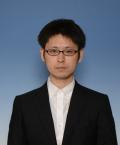### URAMOTO, Takeo

Assistant Professor

My research interests are broadly in the theory of computation; recently, I’m working on intersections between automata theory and number theory. Classically, in automata theory, it has been widely known that there are tight relationships between finite automata, regular languages and finite semigroups (monoids); and via these relationships, one can often prove the decidability of several combinatorial / logical decision problems on regular languages. Such phenomena are in some sense analogous to those known as galois theory where one can characterize when the solutions of an algebraic equation can be expressed by suitable operations (say, four arithmetic operations and roots) in terms of purely group-theoretic properties of the corresponding galois groups (say, solubility). In fact, we can show that this is not just an analogy but actually can be justified in the sense that one can unify galois theory and the classical theory on regular languages and finite semigroups through their categorical axiomatization. Based on this unification, I’m working on intersections between classical class field theory and theory of finite automata; through this research, I’m trying to develop new “semantics” (or applications) of the classical theory of computation.

Keywords Algebraic Language Theory, Theory of Computation, Category Theory, Galois Theory, Class Field Theory Division of Applied Mathematics